# 3.5. SymPy#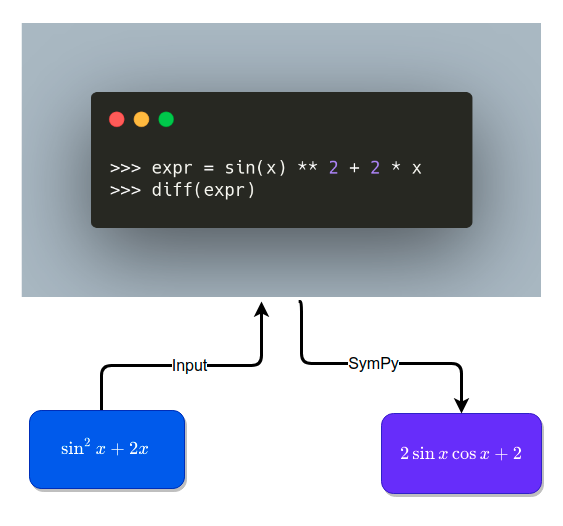## 3.5.1. Motivation#

Have you ever wished to solve a math equation in Python? Wouldn’t it be nice if we could solve an algebraic equation like below in one line of code

eq = (2*x+1)*3*x
solve(eq, x)


Output:

[-1/2, 0]


…or simply work with math symbols instead of boring Python code?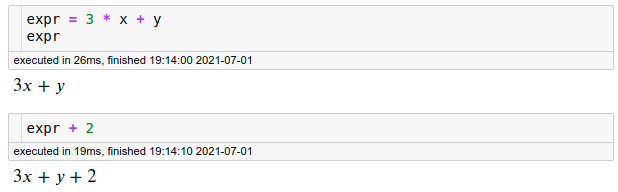That is when SymPy comes in handy.

## 3.5.2. What is SymPy?#

SymPy is a Python library that allows you to compute mathematical objects symbolically.

To install SymPy, type:

pip install sympy


Now let’s go over some of the amazing things that SymPy can do!

from sympy import *


## 3.5.3. Basic Operations#

Normally, when computing a square root, we get a decimal:

18 ** (1 / 2)

4.242640687119285


But with SymPy, we can get a simplified version of the square root instead:

sqrt(18)

$\displaystyle 3 \sqrt{2}$

It is because SymPy tries to represent mathematical objects exactly instead of approximately.

Thus, we will get a fraction instead of a decimal when dividing 2 numbers using SymPy.

25 / 15

1.6666666666666667

frac = Rational(25, 15)
frac

$\displaystyle \frac{5}{3}$

## 3.5.4. Symbols#

The real power of SymPy is its ability to work with symbols. To create symbols, use the method symbols() :

x, y = symbols("x y")
expr = 3 * x + y
expr

$\displaystyle 3 x + y$

Cool! We can create an expression in terms of x and y. What happens if we add a number to this expression?

expr + 2

$\displaystyle 3 x + y + 2$

Aha! + 2 is added to the expression and the expression remains unevaluated.

Why would working with symbols be useful? Because we can now use all kinds of math tricks we learn in school such as expanding, factoring and simplifying an equation to make our life easier.

## 3.5.5. Equations#

### 3.5.5.1. Expand, Factor, and Simplify#

We know that the expansion of the expression on the left is equal to the expression on the right.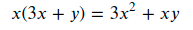Can this be done with SymPy? Yes! SymPy allows us to expand an equation using expand :

expr = x * (3 * x + y)

expansion = expand(expr)
expansion

$\displaystyle 3 x^{2} + x y$

Cool! We can also factor our expression by using factor :

factor(expansion)

$\displaystyle x \left(3 x + y\right)$

Another cool thing we can do with SymPy is to simplify an equation using simplify :

expr = (6 * x ** 2 + 3 * x) / (3 * x)
expr

$\displaystyle \frac{6 x^{2} + 3 x}{3 x}$

Pretty nice, isn’t it?

### 3.5.5.2. Solve an Equation#

One of the most common questions we see when dealing with mathematical symbols is to solve an equation. Luckily, this can also be done with SymPy.

To solve an equation, use solve :

eq = (2 * x + 1) * 3 * x
eq

$\displaystyle x \left(6 x + 3\right)$
solve(eq, x)

[-1/2, 0]


### 3.5.5.3. Substitution#

If we substitute the equation below by 2, what do we get?

$$x(6x+3)$$

We can figure that out using eq.subs(x, 2) :

eq.subs(x, 2)

$\displaystyle 30$

We can also substitute x with another variable to get an expression like below:

eq.subs(x ** 2, 2)

$\displaystyle x \left(6 x + 3\right)$

## 3.5.6. Trigonometric#

Remember all the fun trigonometric identities we learn in high school?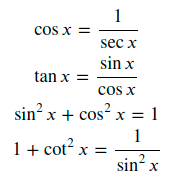Instead of looking up these identities, wouldn’t it be nice if we can use SymPy to figure this out for us?

To simplify expressions using trigonometric identities, use trigsimp()

trigsimp(1 / sec(x))

$\displaystyle \cos{\left(x \right)}$
trigsimp(sin(x) / cos(x))

$\displaystyle \tan{\left(x \right)}$
trigsimp(sin(x) ** 2 + cos(x) ** 2)

$\displaystyle 1$
trigsimp(1 + cot(x) ** 2)

$\displaystyle \frac{1}{\sin^{2}{\left(x \right)}}$

## 3.5.7. Derivatives, Integrals, and Limit#

With SymPy, you can also do calculus! What is the derivative of the expression below?

expr = sin(x) ** 2 + 2 * x
expr

$\displaystyle 2 x + \sin^{2}{\left(x \right)}$

If you cannot figure it out, don’t worry. We can use SymPy to figure it out.

res = diff(expr)
res

$\displaystyle 2 \sin{\left(x \right)} \cos{\left(x \right)} + 2$

Now let’s try to get back to the original expression by taking the integral of the derivate.

integrate(res)

$\displaystyle 2 x + \sin^{2}{\left(x \right)}$

We can also take the limit as x approaches infinity.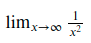limit(
1 / (x ** 2),
x,
oo,
)

$\displaystyle 0$

or 2:

limit(
1 / (x ** 2),
x,
2,
)

$\displaystyle \frac{1}{4}$

## 3.5.8. Special Functions#

SymPy also provides special functions to:

• Find the factorial of a number

factorial(x)

$\displaystyle x!$
factorial(3)

$\displaystyle 6$
• Rewrite the expression in terms of another

tan(x).rewrite(cos)

$\displaystyle \frac{\cos{\left(x - \frac{\pi}{2} \right)}}{\cos{\left(x \right)}}$